# Texas Go Math Grade 3 Lesson 17.2 Answer Key Find Perimeter

Refer to our Texas Go Math Grade 3 Answer Key Pdf to score good marks in the exams. Test yourself by practicing the problems from Texas Go Math Grade 3 Lesson 17.2 Answer Key Find Perimeter.

## Texas Go Math Grade 3 Lesson 17.2 Answer Key Find Perimeter

Unlock the Problem

Find the perimeter of the cover of a notebook.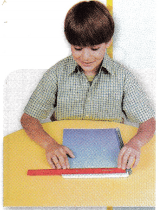Activity Materials; inch ruler

STEP 1:
Estimate the perimeter of a notebook in inches. Record your estimate. inches

STEP 2:
Use an inch ruler to measure the length of each side of the notebook to the nearest inch.

STEP 3:
Record and add the lengths of the sides measured to the nearest inch.
_________ + _________ + ________ + __________ = _________
So, the perimeter of the notebook cover measured to the nearest inch is ____________ inches.
Step 1:Estimated the perimeter of a notebook in inches
8 inch length and 5 inch width
Step 2:Used an inch ruler and measured the length of each side of the notebook to the nearest inch
9 inch lengh and 6 inch width
Step 3: Recorded and added the lengths of the sides measured to the nearest inch
9+6+9+6=30
So, perimeter of the notebook to the nearest inch is 30 inches.

Math Talk
Mathematical Processes

My estimate is very close to the measurement.

Try This! Find the perimeter.

Use an inch ruler to find the length of each side.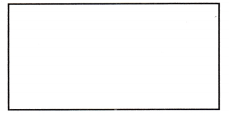Add the lengths of the sides:
________ + ________ + ________ +________ = ________
The perimeter is ________ inches.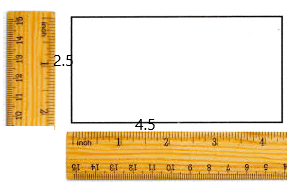Explanation:
Add the lengths of the sides
2.5+4.5+2.5+4.5=14 inches
The perimeter is 14 inches.

Use a centimeter ruler to find the length of each side.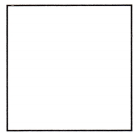Add the lengths of the sides:
________ + ________ + ________ + ________ = ________
The perimeter is ________ centimeters.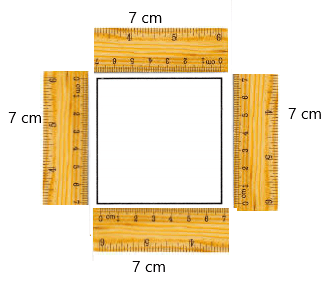Explanation:
Add the lengths of the sides
7+7+7+7=28
The perimeter is 28 centimeters.

Share and Show

Question 1.
Use an inch ruler to find the perimeter of the triangle.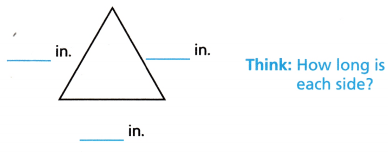___________ inches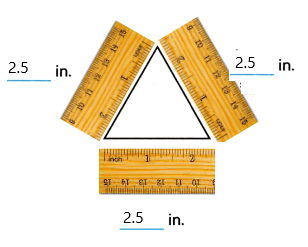Explanation:
Add the lengths of the sides
2.5+2.5+2.5=7.5
The perimeter is 7.5 inches.

Math Talk
Mathematical Processes

Explain how many numbers you add together to find the perimeter of a figure.
We add as many numbers as the number of the sides of the figures to find the perimeter of the figure.

Use a centimeter ruler to find the perimeter.

Question 2.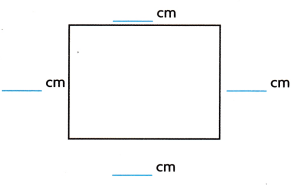_____________ centimetersExplanation:
Add the lengths of the sides
9+7+9+7=32
The perimeter is 32 centimeters.

Question 3._____________ centimetersExplanation:
Add the lengths of the sides
9+7+6+5+2=29
The perimeter is 29 centimeters.

Question 4.
Use the grid paper to draw a figure that has a perimeter of 24 centimeters. Label the length of each side.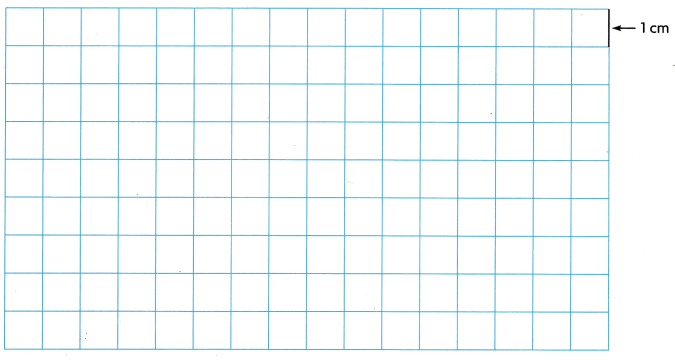Explanation:
I drew a square which has a perimeter of 24 cm.

Problem Solving

Use the photos for 5-6.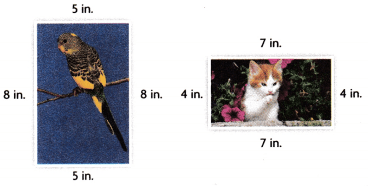Question 5.
Which of the animal photos has a perimeter of 26 inches?
Bird photo has the perimeter of 26 inches

Explanation:
Perimeter of any figure is sum of all its sides.So, add the sides of the photos to find their perimeters
Perimeter of bird photo is 8+5+8+5=26 inches
Perimeter of cat photo is 4+7+4+7=22 inches
Therefore, Bird photo has the perimeter of 26 inches.

Question 6.
Multi-Step Analyze How much greater is the perimeter of the bird photo than the perimeter of the cat photo?
perimeter of the bird photo is 4 inches greater than the perimeter of the cat photo.

Explanation:
Perimeter of any figure is sum of all its sides.So, add the sides of the photos to find their perimeters
Perimeter of bird photo is 8+5+8+5=26 inches
Perimeter of cat photo is 4+7+4+7=22 inches
Subtract 26-22=4 inches
Therefore, perimeter of the bird photo is 4 inches greater than the perimeter of the cat photo.

Question 7.
H.O.T. Multi-Step Erin is putting a fence around her square garden. Each side of her garden is 3 meters long. The fence costs $5 for each meter. How much will the fence cost?Answer: The fence around the Erin’s square garden costs$60.

Explanation:
Erin is putting a fence around her square garden. Each side of her garden is 3 meters long
Perimeter of any figure is sum of all its sides.So, add the sides of the photos to find their perimeters
So, perimeter of the Erin’s square garden is 3+3+3+3=14 meters
The fence costs $5 for each meter.So, multiply 14 meters with$5
14 x 5 = 60
Therefore, The fence around the Erin’s square garden costs $60. Question 8. H.O.T. Write Math Gary’s garden is shaped like a rectangle with two pairs of sides of equal length, and it has a perimeter of 28 feet. Explain how to find the lengths of the other sides if one side measures 10 feet. Answer: Question 9. Evaluate Jill says that finding the perimeter of a figure with all sides of equal length is easier than finding the perimeter of other figures. Do you agree? Explain. Answer: Yes, I agree with jill as all the sides are of equal length we can easily calculate the perimeter by multiplying length of one side with total number of sides of the figure. Daily Assessment Task Fill in the bubble completely to show your answer. Question 10. Sally is putting frosting around the edges of the roof of a gingerbread house. What is the perimeter of the roof?(A) 18 cm (B) 16 cm (C) 8 cm (D) 20 cm Answer: B. 16cm Explanation: perimeter of a figure is sum of all its sides The sum of the sides of the ginger house is 5+3+5+3=16 Therefore, the perimeter of the ginger house is 16cm. Question 11. Kyle is adding a border to his triangular flag. What is the perimeter of the flag?(A) 2 inches (B) 6 inches (C) 3 inches (D) 1 inch Answer: C. 3 inches Explanation: Perimeter of a figure is sum of all its sides The triangular flag is 1 inch all sides 1+1+1 = 3 So, perimeter of flag is 3 inches. Question 12. Multi-Step Pete glues a rope around his rectangular rodeo sign. His sign has side lengths of 2 feet and 3 feet. The rope costs$4 for each foot. How much does Pete pay for rope?
(A) $24 (B)$20
(C) $10 (D)$40
D. $40 Explanation: Pete glues a rope around his rectangular rodeo sign. His sign has side lengths of 2 feet and 3 feet The length around the sign is called as perimeter Perimeter of any figure is sum of all its sides.So, add the sides of the rectangular rodeo sign to find the perimeter 2+3+2+3=10 feet The rope costs$4 for each foot
Multiply 10 with $4 10 x$4 = $14 Therefore, the amount Pete pay for rope is$14.

Texas Test Prep

Question 13.
Austin’s class is making a poster for Earth Day. What is the perimeter of the poster?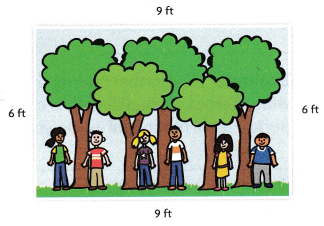(A) 24 feet
(B) 21 feet
(C) 15 feet
(D) 30 feet
D. 30 feet

Explanation:
Perimeter of a figure is sum af all its sides
The lengths if the posters are 9  and 6 feet each
Therefore, Perimeter of the poster is 30 feet.

Texas Go Math Grade 3 Lesson 17.2 Homework and Practice Answer Key

Use a centimeter ruler to find the perimeter.

Question 1.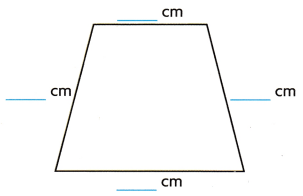____________ centimeters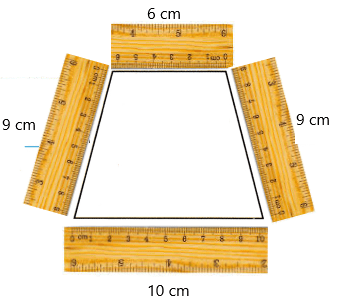Explanation:
Add the lengths of the sides
10+9+9+6=34
The perimeter is 34 centimeters.

Question 2.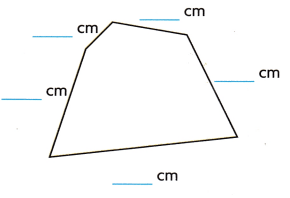____________ centimeters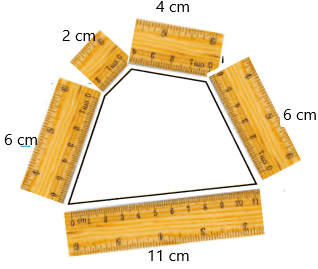Explanation:
Add the lengths of the sides
11+6+6+4+2=29
The perimeter is 29 centimeters.

Problem Solving

Use the drawings for 3-4.

Question 3.
Carly drew quadrilaterals A and B. Which quadrilateral has a perimeter of 28 inches?Explanation:
Perimeter of a figure is sum of all its sides
The perimeter of quadrilateral A is 8+6+8+6= 28 in
The perimeter of quadrilateral B is 8+4+8+4=24 in
Therefore, Quadrilateral A has a perimeter of 28 in.

Question 4.
How much greater is the perimeter of quadrilateral A than the perimeter of quadrilateral B?4 inches

Explanation:
Perimeter of a figure is sum of all its sides
The perimeter of quadrilateral A is 8+6+8+6= 28 in
The perimeter of quadrilateral B is 8+4+8+4=24 in
Subtract perimeter of Quadrilaterl B from A
28-24= 4 in
Therefore, perimeter of quadrilateral A is 4 inches greater than quadrilateral B.

Lesson Check

Question 5.
Benjamin builds a fence in the shape of a triangle. Each side of the fence is the same length. If the perimeter is 36 feet, how long is each side of the fence?
(A) 6 feet
(B) 12 feet
(C) 9 feet
(D) 18 feet
C. 9 feet

Explanation:
Benjamin builds a fence in the shape of a triangle. Each side of the fence is the same length
perimeter of the fence is 36 feet
To find each side of the fence divide 36 feet by 3 as triangle has 3 sided
36 divided by 3 is 9
So, the length of each side of the fence is 9 feet.

Question 6.
Anton puts a rail around his patio. The patio is in the shape of a rectangle with side lengths of 7 feet and 9 feet.
Find the perimeter of the Anton’s patio.
(A) 16 feet
(B) 63 feet
(C) 22 feet
(D) 32 feet
D. 32 feet

Explanation:
Anton puts a rail around his patio. The patio is in the shape of a rectangle with side lengths of 7 feet and 9 feet
Perimeter fo a rectangle is sum of all its sides
The sum of the sides of the rectangular shaped patio is  7+9+7+9=32 feet
Therefore, the perimeter of Anton’s patio is 32 feet.

Question 7.
Alexander makes this name plate from wood in art class.What is the perimeter of the name plate.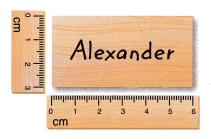(A) 18 cm
(B) 9 cm
(C) 3 cm
(D) 6 cm
A. 18 cm

Explanation:
Alexander makes this name plate from wood in art class
The lengths of the sides are 3 cm and 9 cm
Perimeter of a figure is sum of all its sides
Sum fo the sides of the name plate is 3+6+3+6=18 cm
Therefore, the perimeter of the name plate is 18 cm

Question 8.
Multi-Step Iris sews a border around a blanket. The blanket has side lengths that are 4 feet and 6 feet. The border material costs $2 for each foot. How much does Iris pay for the border. (A)$20
(B) $12 (C)$40
(D) $16 Answer: C.$40

Explanation:
Iris sews a border around a blanket. The blanket has side lengths that are 4 feet and 6 feet
perimeter of a figure is sum of all its sides
Sum of the sides of the blanket is 4+6+4+6=20
The border material costs $2 for each foot Multiply the perimeter fo the blanket with the cost per unit that is 20 feet adn$2
20 ft x $2 =$40
Therefore, Iris pays $40 for the border. Question 9. Multi-Step An artist paints two pictures. Each picture has side lengths of 2 feet and 4 feet. Framing costs$3 for each foot. How much will the artist pay to put a frame around both paintings?
(A) $18 (B)$72
(C) $36 (D)$24
B. $72 Explanation: An artist paints two pictures. Each picture has side lengths of 2 feet and 4 feet. Perimeter of a figure is sum of all its sides So, perimeter of 1 picture is 2+4+2+4= 12 ft Perimeter of 2 picture will be 2 x 12ft = 24 ft Framing costs$3 for each foot
24 ft x $3 =$72